The OpenFOAM FoundationsubCycleTime Class Reference

A class for managing sub-cycling times. More...

Inheritance diagram for subCycleTime: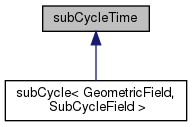[legend]

Public Member Functions

subCycleTime (Time &, const label nSubCycles)
Construct from original time and number of sub-cycles. More...

~subCycleTime ()
Destructor. More...

bool end () const
Return true if the number of sub-cycles has been reached. More...

void endSubCycle ()
End the sub-cycling and reset the time-state. More...

label nSubCycles () const
Return the total number of sub-cycles. More...

label index () const
Return the current sub-cycle index. More...

subCycleTimeoperator++ ()
Prefix increment. More...

subCycleTimeoperator++ (int)
Postfix increment. More...

Detailed Description

A class for managing sub-cycling times.

Source files

Definition at line 48 of file subCycleTime.H.

◆ subCycleTime()

 subCycleTime ( Time & t, const label nSubCycles )

Construct from original time and number of sub-cycles.

Definition at line 30 of file subCycleTime.C.

References Time::subCycle().

Here is the call graph for this function: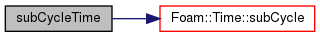◆ ~subCycleTime()

 ~subCycleTime ( )

Destructor.

Definition at line 42 of file subCycleTime.C.

References subCycleTime::endSubCycle().

Here is the call graph for this function: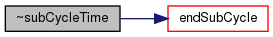◆ end()

 bool end ( ) const

Return true if the number of sub-cycles has been reached.

Definition at line 50 of file subCycleTime.C.

◆ endSubCycle()

 void endSubCycle ( )

End the sub-cycling and reset the time-state.

Definition at line 56 of file subCycleTime.C.

References Time::endSubCycle().

Here is the call graph for this function: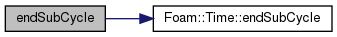Here is the caller graph for this function: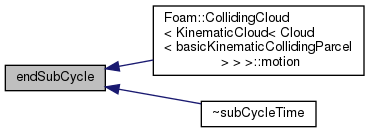◆ nSubCycles()

 label nSubCycles ( ) const
inline

Return the total number of sub-cycles.

Definition at line 78 of file subCycleTime.H.

◆ index()

 label index ( ) const
inline

Return the current sub-cycle index.

Definition at line 84 of file subCycleTime.H.

References subCycleTime::operator++().

Here is the call graph for this function: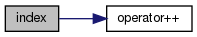◆ operator++() [1/2]

 Foam::subCycleTime & operator++ ( )

Prefix increment.

Definition at line 64 of file subCycleTime.C.

Referenced by subCycleTime::index(), and subCycleTime::operator++().

Here is the caller graph for this function: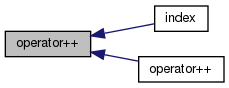◆ operator++() [2/2]

 Foam::subCycleTime & operator++ ( int )

Postfix increment.

Definition at line 72 of file subCycleTime.C.

References subCycleTime::operator++().

Here is the call graph for this function: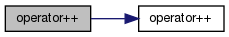The documentation for this class was generated from the following files: